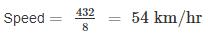# A train is moving at a speed of 72 km/h and covers a certain distance in 6 hours. If the same distance is to be covered in 8 hours, then what will be the speed (in km/h) of the train?a)45b)54c)63d)64Correct answer is option 'B'. Can you explain this answer? Related Test: SSC MTS Mock Test -5

## SSC QuestionSHWETA SHRIVASTAVA Sep 15, 2019
Distance = speed × Time
Let the distance be ‘X’
X = 72 × 6 = 432
Now, same distance is to covered in 8 hours
432 = speed × 8